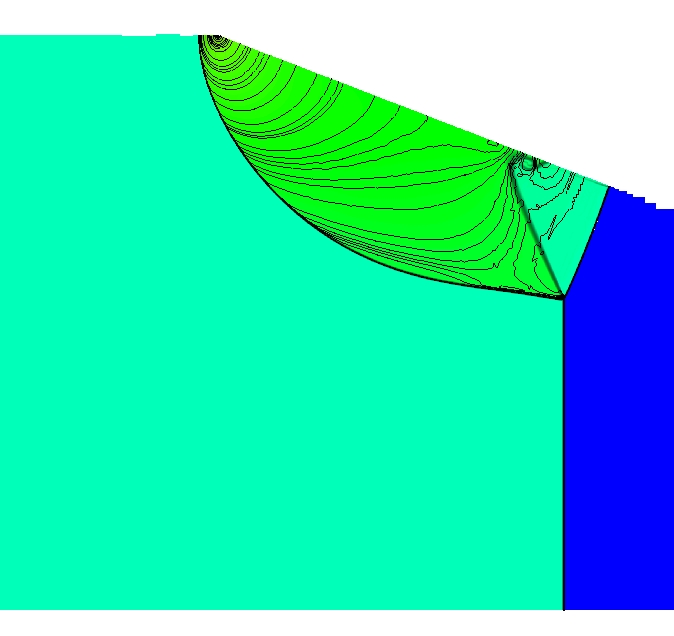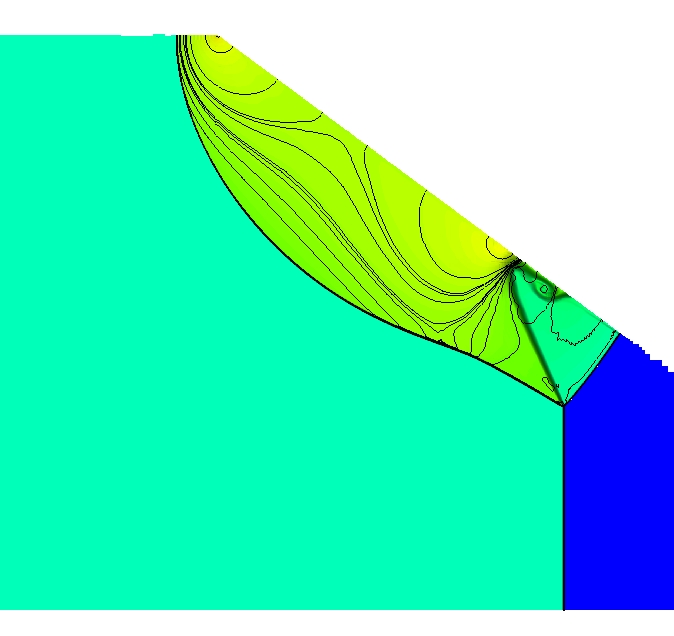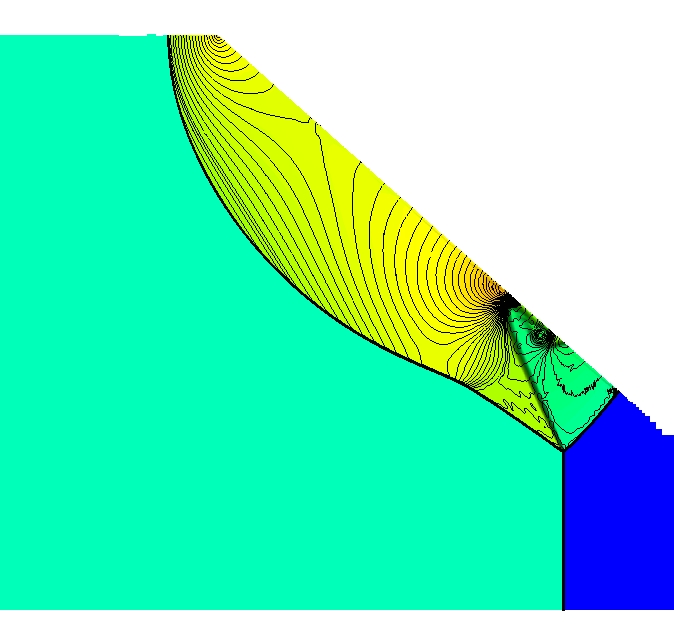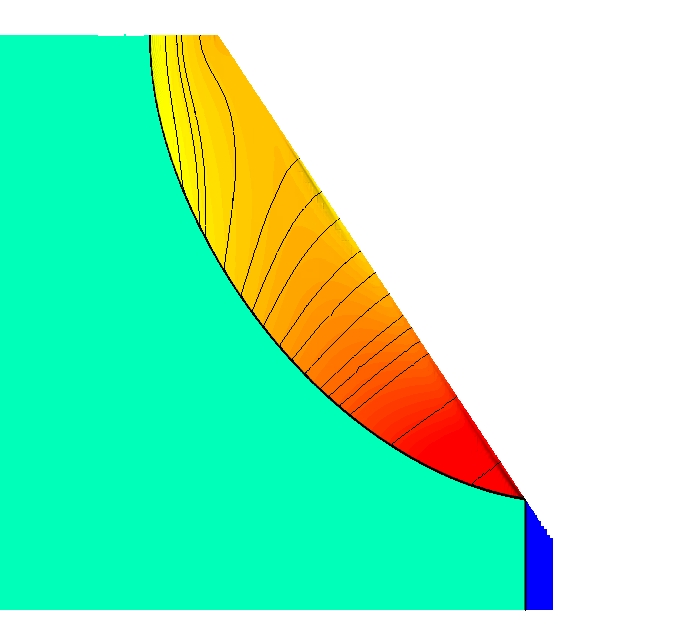Jump:

# Reflected shock structure for conical geometry.

The initial form of the shock reflection as a Mach 6 shock in Argon enters a cone is dependent on the conical half-angle. Here are a series of images all taken at a time when the shock has traveled a distance into the cone equal to 1/3 of the cone dimeter. The full range of reflection behavior expected in a two-dimensional geometry (ie predictions from von Neumann analysis for a shock/wedge interaction) is seen here for the more complicated three-dimensional geometry where the standard analysis is not available.

The full range of reflection behavior from von Neumann reflection, simple Mach reflection, thru double Mach reflection and regular reflection can be seen. Density contours in colour and pressure in lines.

• 15 degree half-angle:• twenty degree half-angle:• twenty five degree half-angle:• thirty five degree half-angle:• forty degree half-angle:• forty five degree half-angle:• fifty degree half-angle:• fifty five degree half-angle:You are here: ConvergingShock > Conical > ConicalStruc

to top